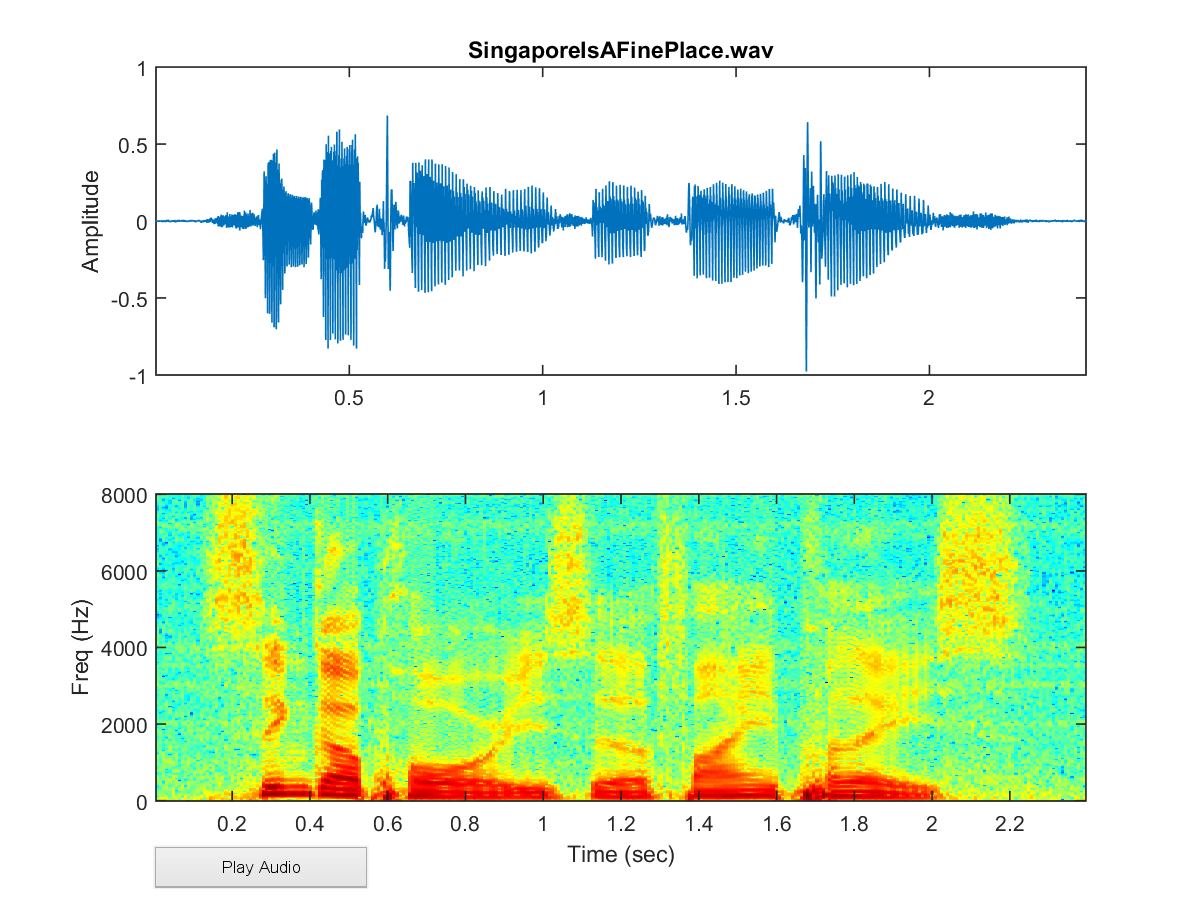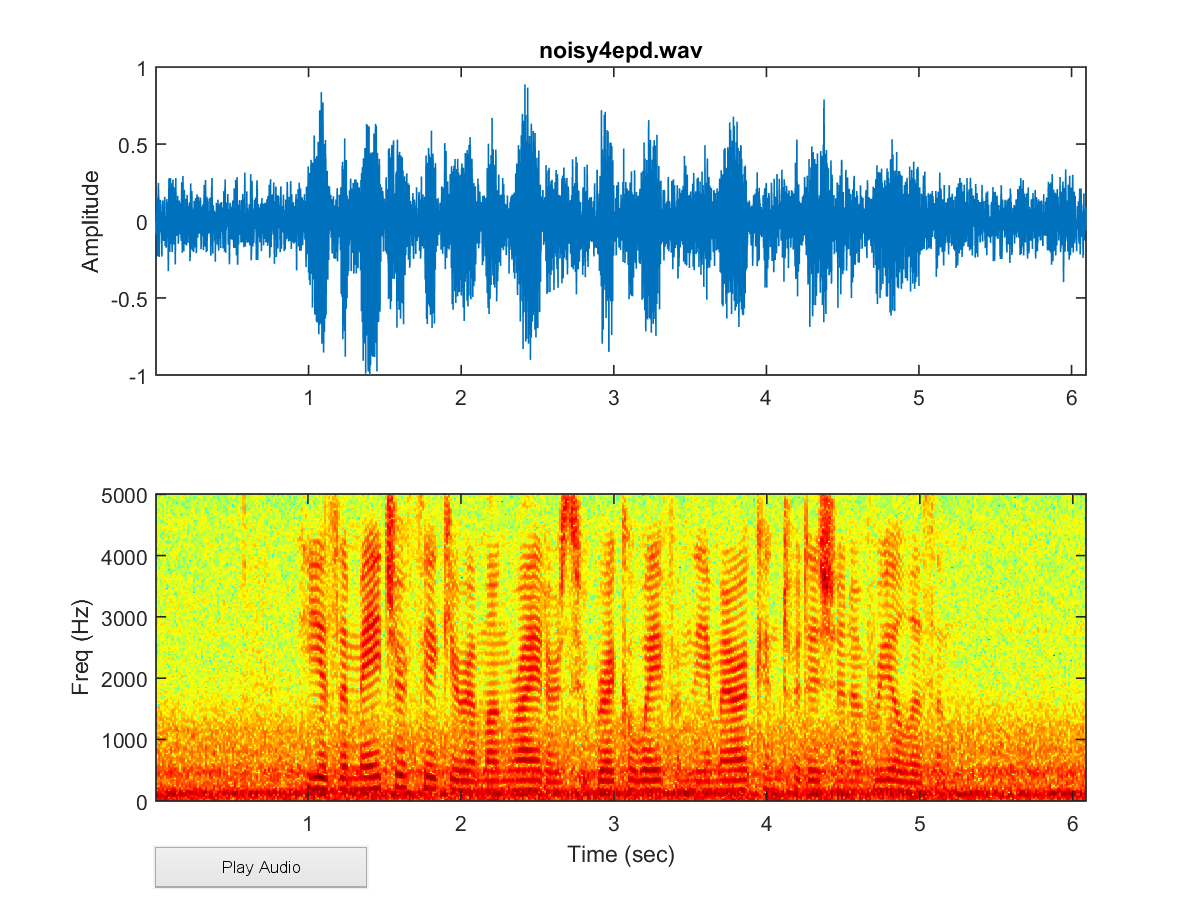## 6-3 EPD in Frequency Domain (嚙踝蕭嚙踝蕭嚙踝蕭嚙踝蕭嚙踝蕭嚙踝蕭嚙踝蕭嚙踝蕭嚙踝蕭嚙踝蕭嚙踝蕭嚙踝蕭嚙踝蕭嚙踝蕭嚙踝蕭)

[chinese][all]

Voiced sounds have harmonic structures in the frequency-domain spectum. Moreover, the energy distribution will be mostly biased toward the low-frequency bands. As a result, we can apply simple mathematical functions on the spectrum for EPD.

If you are not familiar with the definition of spectrum (or more specifically, amplitude spectrum), you do not need to worry about this at this stage. All you need to know is that the amplitude spectrum is the distribution of energy with respective to frequency. More information about the spectrum and its mathematical definition will be covered in later chapters. Here are two examples to show spectra of audio signals:

Example 1: epdShowSpec01.mwaveFile='SingaporeIsAFinePlace.wav'; au=myAudioRead(waveFile); time=(1:length(au.signal))/au.fs; subplot(211); plot(time, au.signal); axis([min(time), max(time), -1, 1]); ylabel('Amplitude'); title(waveFile); subplot(212); frameSize=256; overlap=frameSize/2; [S,F,T]=spectrogram(au.signal, frameSize, overlap, 4*frameSize, au.fs); magSpec=abs(S); specgram=log(magSpec); imagesc(T, F, specgram); axis xy xlabel('Time (sec)'); ylabel('Freq (Hz)'); audioPlayButton(au); colormap jet;Example 2: epdShowSpec02.mwaveFile='noisy4epd.wav'; au=myAudioRead(waveFile); time=(1:length(au.signal))/au.fs; subplot(211); plot(time, au.signal); axis([min(time), max(time), -1, 1]); ylabel('Amplitude'); title(waveFile); subplot(212); frameSize=256; overlap=frameSize/2; [S,F,T]=spectrogram(au.signal, frameSize, overlap, 4*frameSize, au.fs); magSpec=abs(S); specgram=log(magSpec); imagesc(T, F, specgram); axis xy xlabel('Time (sec)'); ylabel('Freq (Hz)'); audioPlayButton(au); colormap jet;Audio Signal Processing and Recognition (音訊處理與辨識)